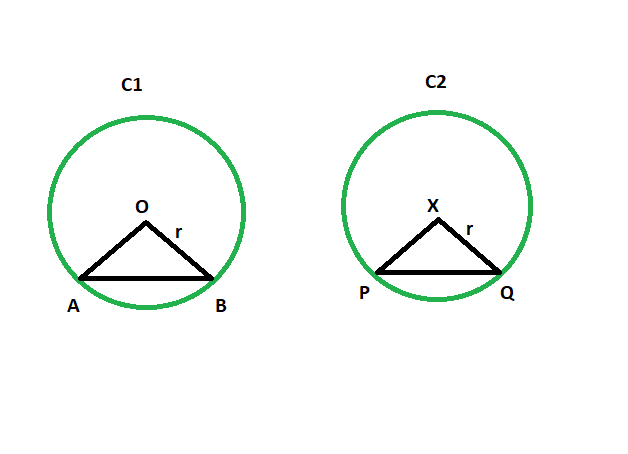Skip to content
Related Articles
Angle subtended by the chord to center of the circle when the angle subtended by the another equal chord of a congruent circle is given
• Last Updated : 10 Mar, 2021

Given are two congruent circles with two equal chords. The angle subtended to the centre from the chord of one of the circles is given. The task is to find the angle subtended by the chord to the centre of another circle.
Examples:

```Input: z = 48
Output: 48 degrees

Input: z = 93
Output: 93 degrees```Approach

• In triangle AOB and PXQ

```AO = PX(radii of congruent circles)
BO = QX(radii of congruent circles)
AB = PQ(equal chords)```
•
• So, triangle AOB is congruent with triangle PXQ

• So, angle AOB = angle PXQ

Equal chords of congruent circles subtend equal angles at their centres.

Below is the implementation of the above approach:

## C++

 `// C++ program to find the angle subtended by the chord``// to the centre of the circle when the angle subtended``// by another equal chord of a congruent circle is given` `#include ``using` `namespace` `std;` `void` `anglequichord(``int` `z)``{``    ``cout << ``"The angle is "` `<< z``         ``<< ``" degrees"` `<< endl;``}` `// Driver code``int` `main()``{``    ``int` `z = 48;``    ``anglequichord(z);``    ``return` `0;``}`

## Java

 `// Java program to find the angle subtended by the chord``// to the centre of the circle when the angle subtended``// by another equal chord of a congruent circle is given` `import` `java.io.*;` `class` `GFG``{``    ` `static` `void` `anglequichord(``int` `z)``{``    ``System.out.println (``"The angle is "` `+ z + ``" degrees"``);``}` `// Driver code``public` `static` `void` `main (String[] args)``{` `    ``int` `z = ``48``;``    ``anglequichord(z);` `}``}`

## Python 3

 `# Python 3 program to find the angle subtended by the chord``# to the centre of the circle when the angle subtended``# by another equal chord of a congruent circle is given`  `def` `anglequichord(z):``    ``print` `(``"The angle is "` `, z``        ``, ``" degrees"``)` `# Driver code``if` `__name__ ``=``=` `"__main__"``:``    ``z ``=` `48``    ``anglequichord(z)` `# This code is contributed by ChitraNayal`

## C#

 `// C# program to find the angle subtended by the chord``// to the centre of the circle when the angle subtended``// by another equal chord of a congruent circle is given``using` `System;` `class` `GFG``{``    ` `    ``static` `void` `anglequichord(``int` `z)``    ``{``        ``Console.WriteLine(``"The angle is "` `+ z + ``" degrees"``);``    ``}``    ` `    ``// Driver code``    ``public` `static` `void` `Main ()``    ``{``    ` `        ``int` `z = 48;``        ``anglequichord(z);``    ``}``}` `// This code is contributed by AnkitRai01`

## Javascript

 ``
Output:
`The angle is 48 degrees`

Attention reader! Don’t stop learning now. Get hold of all the important DSA concepts with the DSA Self Paced Course at a student-friendly price and become industry ready.  To complete your preparation from learning a language to DS Algo and many more,  please refer Complete Interview Preparation Course.

In case you wish to attend live classes with industry experts, please refer DSA Live Classes

My Personal Notes arrow_drop_up